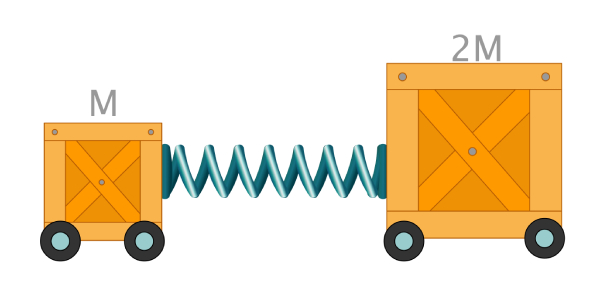# Linear Motion Quiz Questions And Answers

20 Questions | Attempts: 3165
ShareSettingsDo you understand the term linear motion in Physics? Try out this linear motion quiz with questions and answers. It is an important topic that needs to be covered if you are into learning Physics. We have got this practice test for you to know how well you understand linear motion. Your scores will reveal your knowledge and understanding of this topic. Go for it and try getting a perfect score. All the best! Do share the quiz with others interested in Physics.

• 1.
On a position-time graph, a changing velocity is represented by a straight line.
• A.

True

• B.

False

• 2.
Calculate the distance (in km) that Charlie runs if he maintains an average speed of 8 km/hr for 1 hr.
• 3.
Speed is
• A.

A measure of how fast something is moving.

• B.

The distance covered per unit time.

• C.

Always measured in terms of a unit of distance divided by a unit of time.

• D.

All of the above

• 4.
Calculate the distance you will travel if you maintain an average speed of 10 m/s for 40 seconds.
• 5.
Acceleration describes
• A.

Any change in velocity.

• B.

An object that is speeding up.

• C.

An object that is slowing down.

• D.

An object that is changing direction.

• E.

All of the above

• 6.
Calculate the speed of a bowling ball that moves 8 meters in 4 seconds.
• 7.
As a ball falls freely, the distance it falls each second is the same.
• A.

True

• B.

False

• 8.
Calculate the acceleration of a bus that goes from 10 km/h to a speed of 50 km/h in 10 seconds.
• 9.
If an object moves from one position to another, it is
• A.

Accelerating

• B.

Moving

• C.

Speeding

• D.

In free fall

• 10.
Calculate the speed (in m/s) of a skateboarder who accelerates from rest for 3 seconds down a ramp at an acceleration of 5 m/s2.
• 11.
Calculate the vertical distance an object dropped from rest would cover in 12 seconds if it fell freely without air restistance.
• 12.
An apple drops from a tree and hits the ground in 1.5 seconds. Calculate how far it falls.
• 13.
Calculate the acceleration of a ball that starts from rest and rolls down a ramp and gains a speed of 25 m/s in 5 seconds.
• 14.
Objects in free fall are only influenced by gravity, not by air resistance.
• A.

True

• B.

False

• 15.
Calculate the instantaneous speed (in m/s) at the 10-second mark for a car that accelerates at 2 m/s2 from a position of rest.
• 16.
Elapsed time is the time that passes from the beginning to the end of a fall.
• A.

True

• B.

False

• 17.
Calculate your average walking speed when you step 1 meter in 0.5 seconds.
• 18.
When you look at the speedometer in a moving car, you can see the car's
• A.

Instantaneous speed

• B.

Average speed

• C.

Instantaneous acceleration

• D.

Average acceleration

• E.

Average distance traveled

• 19.
Suppose you take a trip that covers 240 km and takes 4 hours. Your average speed is
• A.

480 km/h.

• B.

240 km/h.

• C.

120 km/h.

• D.

60 km/h.

• 20.
A position-time graph of constant velocity will show a
• A.

Straight line.

• B.

Curved line.

• C.

Wavy line

• D.

Distorted line

## Related TopicsBack to top
×

Wait!
Here's an interesting quiz for you.